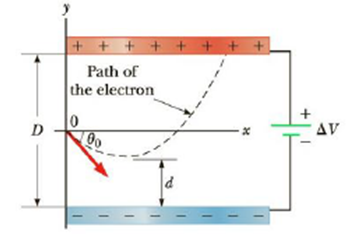Chapter 16, Problem 72AP

Chapter
Section
Textbook Problem

An electron is fired at a speed v0 = 5.6 × 106 m/s and at an angle θ0 = −45° between two parallel conducting plates that are D = 2.0 mm apart, as in Figure P16.72. If the voltage difference between the plates is ΔV = 100. V, determine (a) how close, d, the electron will get to the bottom plate and (b) where the electron will strike the top plate.Figure P16.72

(a)

To determine
The distance of closest approach.

Explanation

Given info: The speed of electron ( v0 ) is 5.6×106m/s . The distance between the plates (D) is 2.0 mm. The potential difference between the plates ( ΔV ) is 100 V.

Explanation:

The electric field strength is,

E=ΔVD

The acceleration of electron is,

a=qEm

Formula to calculate the distance of closest approach is,

d=D2(v0sinθ)22a

y=D2mv02Dsin2θ2q(ΔV)

Substitute 9.11×1031kg for m, 2.0 mm for D, 5.6×106m/s for v0 , 45ο for θ , 100 V for ΔV and 1

(b)

To determine
The horizontal displacement of the electron.

Still sussing out bartleby?

Check out a sample textbook solution.

See a sample solution

The Solution to Your Study Problems

Bartleby provides explanations to thousands of textbook problems written by our experts, many with advanced degrees!

Get Started